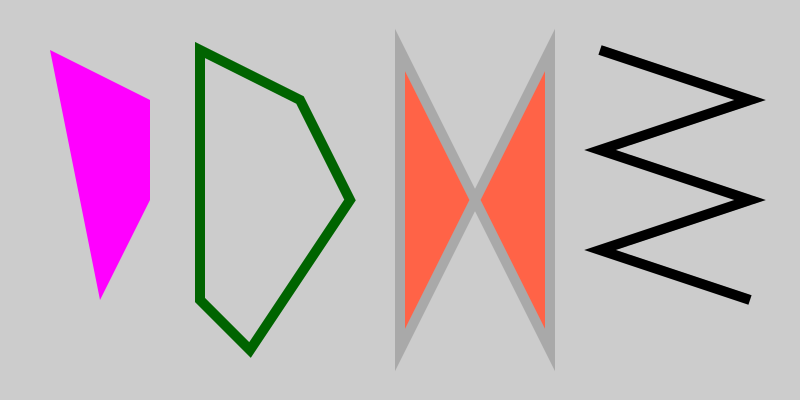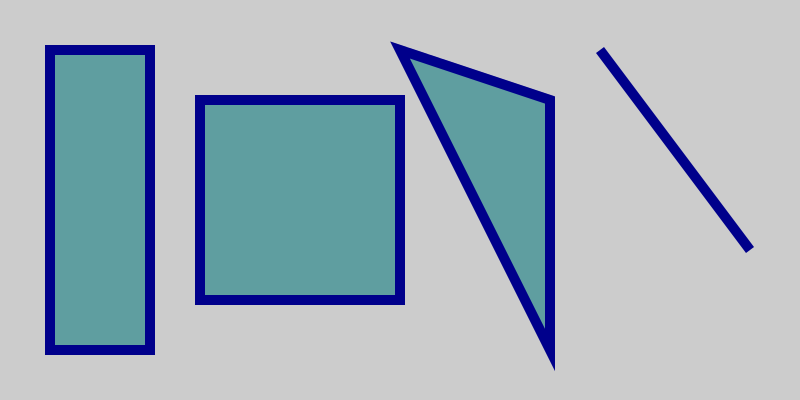# Polygons in generativepy

Martin McBride, 2020-10-07
Tags generativepy tutorial fill stroke rectangle square polygon
Categories generativepy generativepy tutorial

This tutorial shows how to create polygons in generativepy. You should read through the fill and stroke tutorial first, if you haven't already.

## Polygon example code

In this section we will see how to polygons, by filling, stroking, or both filling and stroking. We will also see an example of a self intersecting shape, and an open shape:

from generativepy.drawing import make_image, setup
from generativepy.color import Color
from generativepy.geometry import Polygon, Rectangle, Square, Triangle, Line

def draw(ctx, width, height, frame_no, frame_count):
setup(ctx, width, height, width=800, background=Color(0.8))

Polygon(ctx).of_points(((50, 50), (150, 100),\
(150, 200), (100, 300)))\
.fill(Color('magenta'))

Polygon(ctx).of_points(((200, 50), (200, 300),\
(250, 350), (350, 200),\
(300, 100)))\
.stroke(Color('darkgreen'), 10)

Polygon(ctx).of_points(((400, 50), (550, 350),\
(550, 50), (400, 350)))\
.fill(Color('tomato'))\
.stroke(Color('darkgrey'), 10)

Polygon(ctx).of_points(((600, 50), (750, 100),\
(600, 150), (750, 200),\
(600, 250), (750, 300)))\
.open()\
.stroke(Color('black'), 10)

make_image("polygons.png", draw, 800, 400)


Here is the resulting image:This section of the code draws the leftmost shape (a filled, magenta quadrilateral):

    Polygon(ctx).of_points(((50, 50), (150, 100),\
(150, 200), (100, 300)))\
.fill(Color('magenta'))


The of_points takes a single argument, a sequence of (x, y) points. In this case, we are using a tuple containing four (x, y) tuples, like this:

((50, 50), (150, 100), (150, 200), (100, 300))


Notice that this starts and ends with two brackets, so when we pass this into a function call we need triple brackets, and shown in the example code.

We then fill the shape with magenta.

The second shape is drawn like this:

    Polygon(ctx).of_points(((200, 50), (200, 300),\
(250, 350), (350, 200),\
(300, 100)))\
.stroke(Color('darkgreen'), 10)


This creates a pentagon. We stroke it in green, with a width of 10 pixels, but we don't fill it.

The third shape is drawn like this:

    Polygon(ctx).of_points(((400, 50), (550, 350),\
(550, 50), (400, 350)))\
.fill(Color('tomato'))\
.stroke(Color('darkgrey'), 10)


This shape is a quadrilateral, but it is drawn so that some of the edges cross over - it is self intersection. We fill it and stroke it. The fill covers both the enclosed parts of the shape.

The final, rightmost shape is drawn like this:

    Polygon(ctx).of_points(((600, 50), (750, 100),\
(600, 150), (750, 200),\
(600, 250), (750, 300)))\
.open()\
.stroke(Color('black'), 10)


The open call makes it an open polygon - it is drawn in the usual way, but the final point is not connected back to the start point.

## Using polygon shape objects

There are several shapes that can be used to create specific polygons (such as squares). You can do this using the general Polygon object, but these additional objects are sometimes convenient. Here is the code:

from generativepy.drawing import make_image, setup
from generativepy.color import Color
from generativepy.geometry import Rectangle, Square, Triangle, Line

def draw(ctx, width, height, frame_no, frame_count):
setup(ctx, width, height, width=800, background=Color(0.8))

Rectangle(ctx).of_corner_size((50, 50), 100, 300)\
.stroke(Color('darkblue'), 10)

Square(ctx).of_corner_size((200, 100), 200)\
.stroke(Color('darkblue'), 10)

Triangle(ctx).of_corners((400, 50), (550, 350),\
(550, 100))\
.stroke(Color('darkblue'), 10)

Line(ctx).of_start_end((600, 50), (750, 250))\
.stroke(Color('darkblue'), 10)

make_image("poly-shapes.png", draw, 800, 400)


Here are the objects we use:

• Rectangle draws a rectangle based on the position of its top left corner (50, 50), its width 100 and its height 300.
• Square draws a square based on the position of its top left corner (200, 100), and the length of its side 200.
• Triangle draws a triangle based on the positions of its three corners.
• Line draws a line based on the position of its start and end points.

All the shapes are filled and stroked, except the line which is only stroked (a line doesn't contain an area, so filling it would have no effect).

This is the result: